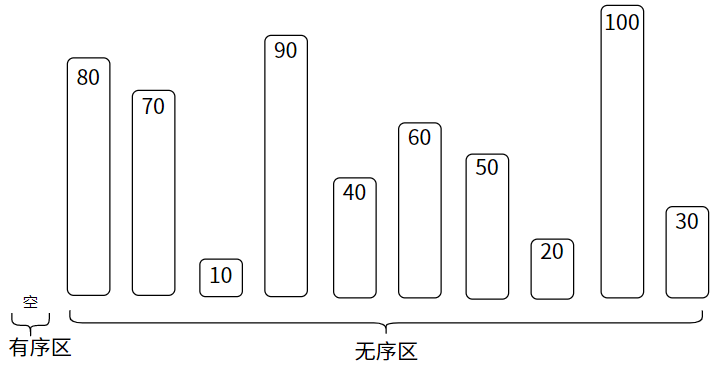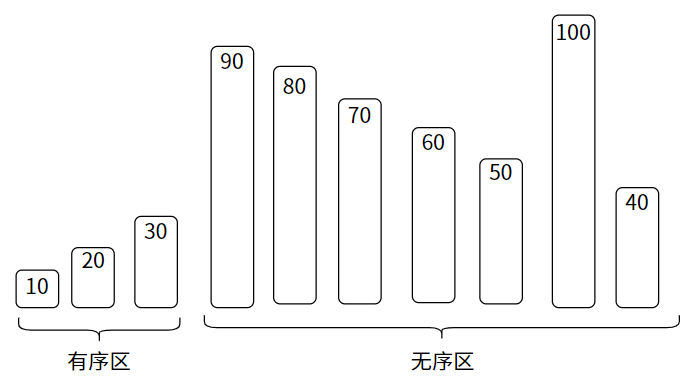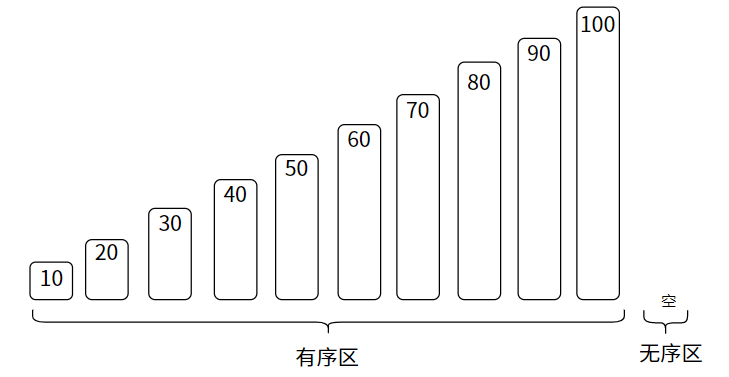9. 排序（Sort）## 9.0. 什么是排序？

``````/*一个交换函数，交换数组中下标为i和j的元素位置*/
void swap(int *array, int i, int j)
{
int tmp = array[i];
array[i] = array[j];
array[j] = tmp;
}``````

• 只对数组排序，并约定数组长度 `length = 10`
• 全部排序算法均按升序排序
• 由于是针对数组，所以关键字就是其元素值本身。比较关键字即是比较元素值，不再区分。

## 9.1. 暴力排序（Violent Sort）

• 变量 `i`：某个元素的下标；
• 变量 `j`：下标 `i` 之后的下标。

• 外层循环控制轮数；
• 内层循环控制每轮的比较和交换。``````/* 暴力排序
* array : 数组
* length : 数组长度
*/
void violent_sort(int *array, int length)
{
int i, j;
// 外层循环，遍历数组，控制轮数
for (i = 0; i < length; i++) {
// 内层循环，循环比较 array[i] 和其之后的元素，控制循环次数
for (j = i + 1; j < length; j++) {
if (array[i] > array[j]) { // 如果大于则交换
swap(array, i, j);
}
}
}
}``````

## 9.2. 冒泡排序（Bubble Sort）

• 变量 `i`：用于控制轮数；

• 变量 `j`：元素下标，`j+1` 为其相邻元素的下标。

• 外层循环控制轮数
• 内层循环控制每轮所有相邻元素的比较和交换``````/* 冒泡排序
* array : 数组
* length : 数组长度
*/
void bubble_sort(int *array, int length)
{
int i, j;
// 外层循环，控制轮数。每一轮排好一个元素
for (i = 0; i < length; i++) {
// 内层循环，循环比较数组中未排序的元素
for (j = 0; j < length - i - 1; j++) {
// 若前者大于后者则交换
if (array[j] > array[j + 1]) {
swap(array, j, j + 1);
}
}
}
}``````

`unsorted` 通过记录在某轮排序中是否发生了交换，如果没发生，就意味着已经有序。

``````void bubble_sort_v3(int *array, int length)
{
int i, j;
int unsorted = 1; // 标识变量
for (i = 0; i < length && unsorted; i++) {
unsorted = 0;
for (j = 0; j < length - i - 1; j++) {
if (array[j] > array[j + 1]) {
swap(array, j, j + 1);
unsorted = 1; // 发生交换，说明尚未有序
}
}
}
}``````

## 9.3. 简单选择排序（Simple Selection Sort）

• 变量 `i`：基准元素的下标；
• 变量 `j`：基准元素之后的元素的下标；
• 变量 `min`：找到的最小元素的下标。

• 外层循环用于控制轮数；
• 内层循环用于控制每轮的若干次比较和最多一次的交换。``````/*
* 简单选择排序
* array : 数组
* length : 数组长度
*/
void simple_selection_sort(int *array, int length)
{
int i, j, min;
for (int i = 0; i < length - 1; i++) {
min = i; // 最小元素初始化为基准元素
// 找最小元素
for (int j = i + 1; j < length; j++) {
if (array[min] > array[j]) {
min = j;
}
}
// 在基准元素之后的元素中找到了比基准元素小的值
if (i != min) {
swap(array, i, min); // 交换
}
}
}``````

## 9.4. 直接插入排序（Straight Insertion Sort）``````/*
* 直接插入排序
* array : 数组
* length : 数组长度
*/
void straight_insertion_sort(int *array, int length)
{
int i, j;
// 外层循环 决定待插入值
for (i = 1; i < length; i++) {
if (array[i] < array[i - 1]) {
int tmp = array[i]; // 待插入值
// 内层循环 在有序区中为待插入值腾出位置
for (j = i - 1; j >= 0 && array[j] > tmp; j--) {
array[j + 1] = array[j];
}
array[j + 1] = tmp; // 插入
}
}
}``````22
Lv1
……

16

6

3